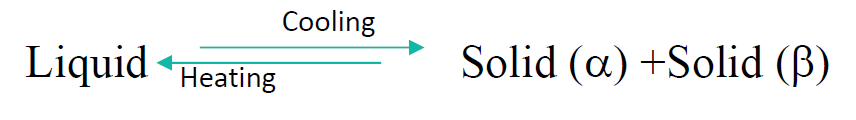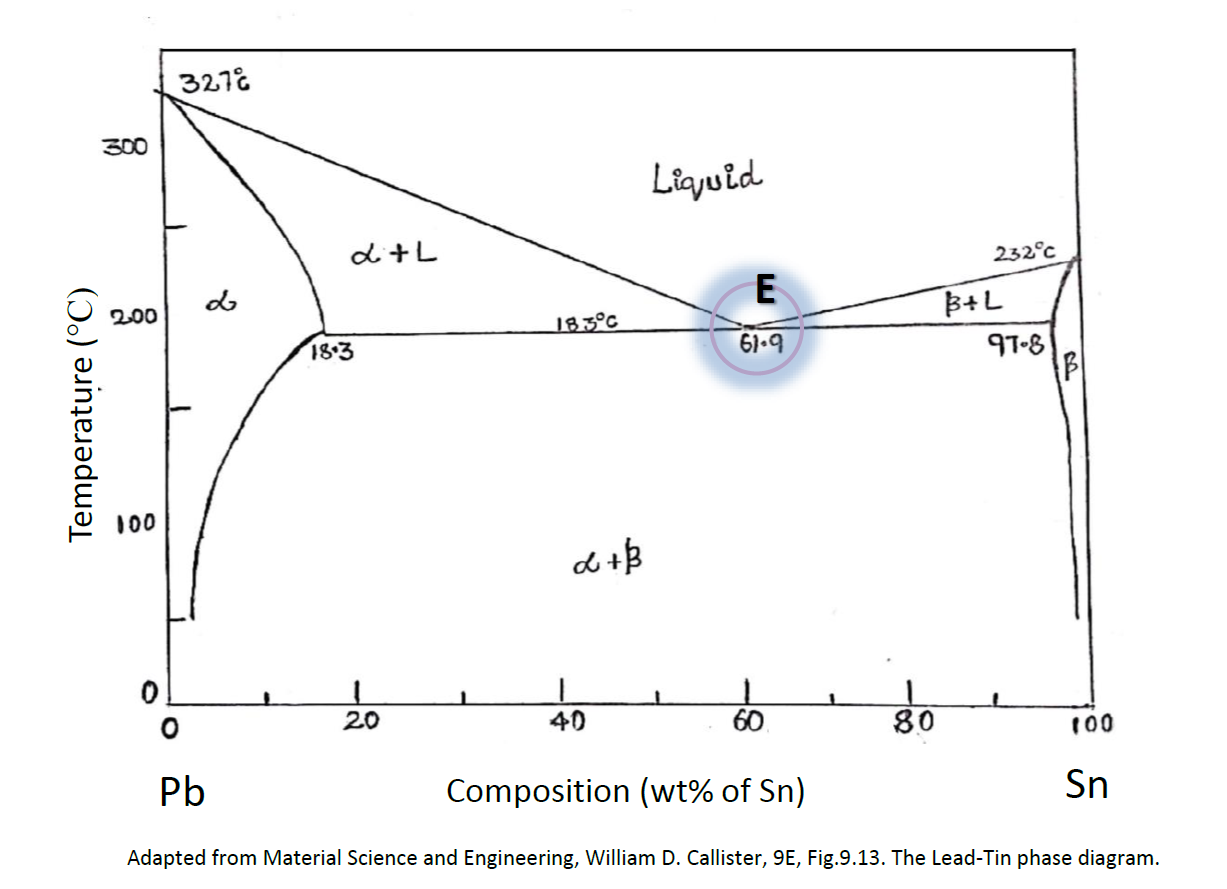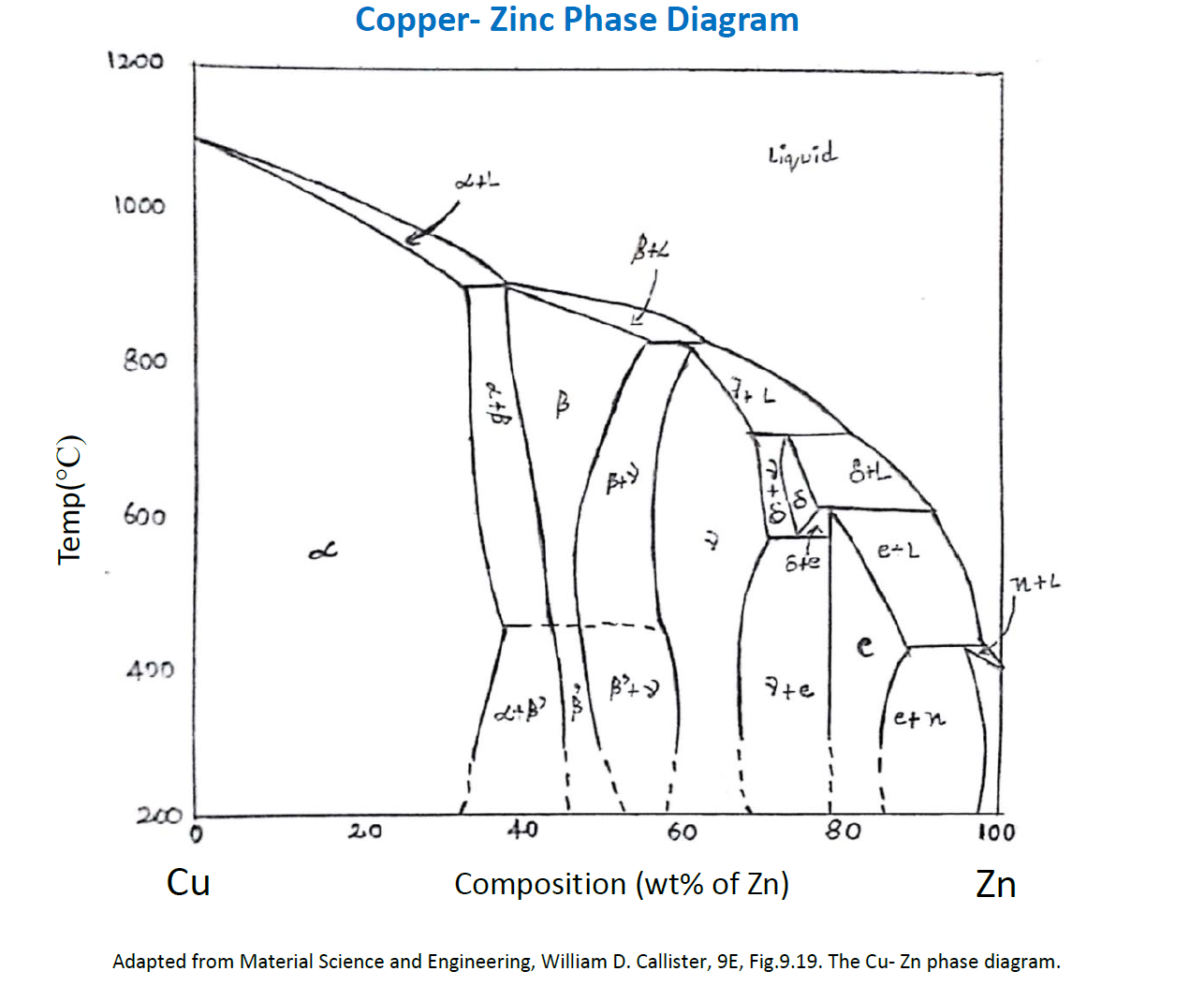### Eutectic system

A eutectic is mixture of more than 2 phases at a given composition that having least melting point. It is the system where the phases crystallize from molten solution. The term comes from the Greek 'eutektos', meaning 'easily melted‘.
In eutectic system, the two elements are completely soluble in the liquid phase but,
Completely insoluble in the solid phase. (Bismuth and Cadmium)   or
Partially soluble in the solid phase. (Lead and Tin)

Completely soluble in the liquid phase but completely insoluble in the solid Phase.Here is a phase diagram of lead and tin indicating point E which is a eutectic point of the alloy. At this alloy composition of 61.9 wt % , with the increase or decrease in temperature the phase directly change from solid(a) +solid(b) to liquid or vice versa without passing through any intermediate phase .
The eutectic reaction involves three phases at eutectic point which is also the equilibrium of phase diagram.

Therefore,
Number of phases = 3

Number of components = 2 (Pb and Sn)

Applying Gibbs phase rule we have,
P + F = C + 1(Number of non-compositional variable)
F (Degree of freedom) = 2+1-3=> 0

This means that the composition of all the 3 phases along with the 183°C are fixed and defined which in fact sufficient to determine the characteristics of the alloy at eutectic point.

Let us consider a point in the phase diagram where both solid as well as liquid phase is present.
Now, Number of phases at that point = 2
Number of components = 2 (Pb and Sn)
Applying Gibbs phase rule we have,
P + F = C + 1(Number of non-compositional variable)
F (Degree of freedom) = 2+1-2 => 1
Hence it is necessary to specify any 1 of the phases to accurately put up the system.The copper –Zinc system is termed as intermediate solid solution or intermediate phase because it contains more than two solid solutions.

There are in total 6 different solid solutions, in which two are terminal i.e. and  and  remaining 4 intermediates are , and e. The ’ phase is noted as solid solution, in which the Cu and Zn atoms are present in a specific ordered arrangement within one each unit cell. Few phase boundary lines are dashed at the bottom which justifies that their positions have not been determined exactly.
This is because at lower temperatures, the rate of diffusion is slow, and relatively longer time is required to reach the equilibrium. For different regions in the phase diagram the composition, phase and amount of phases are determined by the same Tie line and Lever rule.

The commercially available Brasses are Cu-Zn alloys.
Example: The cartridge Brass composition is 70 wt% of Copper and 30 wt% of Zinc